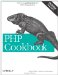# Section 2.0. Introduction

### 2.0. Introduction

In everyday life, numbers are easy to identify. They're 3:00 P.M., as in the current time, or \$1.29, as in the cost of a pint of milk. Maybe they're like Π, the ratio of the circumference to the diameter of a circle. They can be pretty large, like Avogadro's number, which is about 6 x 1023. In PHP, numbers can be all these things.

However, PHP doesn't treat all these numbers as "numbers." Instead, it breaks them down into two groups: integers and floating-point numbers. Integers are whole numbers, such as -4, 0, 5, and 1,975. Floating-point numbers are decimal numbers, such as -1.23, 0.0, 3.14159, and 9.9999999999.

Conveniently, most of the time PHP doesn't make you worry about the differences between the two because it automatically converts integers to floating-point numbers and floating-point numbers to integers. This conveniently allows you to ignore the underlying details. It also means 3/2 is 1.5, not 1, as it would be in some programming languages. PHP also automatically converts from strings to numbers and back. For instance, 1+"1" is 2.

However, sometimes this blissful ignorance can cause trouble. First, numbers can't be infinitely large or small; there's a minimum size of 2.2e-308 and a maximum size of about 1.8e308.[] If you need larger (or smaller) numbers, you must use the BCMath or GMP libraries, which are discussed in Recipe 2.14.

[] These numbers are actually platform-specific, but the values are common because they are from the 64-bit IEEE standard 754.

Next, floating-point numbers aren't guaranteed to be exactly correct but only correct plus or minus a small amount. This amount is small enough for most occasions, but you can end up with problems in certain instances. For instance, humans automatically convert 6 followed by an endless string of 9s after the decimal point to 7, but PHP thinks it's 6 with a bunch of 9s. Therefore, if you ask PHP for the integer value of that number, it returns 6, not 7. For similar reasons, if the digit located in the 200th decimal place is significant to you, don't use floating-point numbers'instead, use the BCMath and GMP libraries. But for most occasions, PHP behaves very nicely when playing with numbers and lets you treat them just as you do in real life.PHP Cookbook: Solutions and Examples for PHP Programmers
ISBN: 0596101015
EAN: 2147483647
Year: 2006
Pages: 445

Similar book on Amazon